# Chemistry Science Paper 2 2015-2016 ICSE Class 10 Question Paper Solution

Chemistry [Science Paper 2]
Date & Time: 18th March 2016, 11:00 am
Duration: 2h

Question 1 is Compulsory.

Attempt any four from Question 2 to Question 7

 1
 1.1

Metals are good _____________ (oxidizing agents / reducing agents) because they are electron __________ (acceptors / donors).

Concept: Types of Elements: Non-metal
Chapter: [0.07] Metallurgy

Electrovalent compounds have ________________ (high / low) melting points.

Concept: Electrovalent (or Ionic) Bond
Chapter: [0.02] Chemical Bonding

Higher the pH value of a solution, the more ____________ (acidic / alkaline) it is.

Concept: Types of Elements: Metals
Chapter: [0.07] Metallurgy

_____________ (AgCl / PbCl2), a white precipitate is soluble in excess NH4OH

Concept: Action of Alkalis on Metal Oxides
Chapter: [0.04] Analytical Chemistry

Conversion of ethane to ethane is an example of ____________ (hydration / hydrogenation).

Concept: Ethanol
Chapter: [0.09] Organic Chemistry
 1.2

Choose the correct answer from the options given below

An element with the atomic number 19 will most likely combine chemically with the element whose atomic number is:

(A) 17

(B) 11

(C) 18

(D) 20

Concept: Periodic Properties
Chapter: [0.01] Periodic Table, Periodic Properties and Variations of Properties

Choose the correct answer from the options given below:

The ratio between the number of molecules in 2g of hydrogen and 32g of oxygen is:

[Given that H = 1, O = 16]

1: 2

1: 0.01

1: 1

0.01: 1

Concept: Mole Concept
Chapter: [0.05] Mole Concept and Stoichiometry

Choose the correct answer from the options given below :

The two main metals in Bronze are:

(A) Copper and zinc

(C) Copper and nickel

(D) Copper and tin

Concept: Types of Elements: Non-metal
Chapter: [0.07] Metallurgy

Choose the correct answer from the options given below :

The particles present in strong electrolytes are :

(A) Only molecules

(B) Mainly ions

(C) Ions and molecules

(D) Only atoms

Concept: Electrolysis
Chapter: [0.06] Electrolysis

Choose the correct answer from the options given below :

The aim of the Fountain experiment is to prove that :

(A) HCl turns blue litmus red

(B) HCl is denser than air

(C) HCl is highly soluble in water

(D) HCl fumes in moist air

Concept: Physical Properties of Hydrogen Chloride Gas
Chapter: [0.081] Hydrogen Chloride
 1.3

Write balanced chemical equations for Action of warm water on AIN.

Concept: Preparation of Aqueous Ammonia
Chapter: [0.08199999999999999] Ammonia

Write balanced chemical equations for Action of hot and concentrated Nitric acid on copper

Concept: Laboratory Preparation of Nitric Acid
Chapter: [0.083] Nitric Acid

Write balanced chemical equations for Action of Hydrochloric acid on sodium bicarbonate.

Concept: Laboratory Preparation of Hydrogen Chloride Gas
Chapter: [0.081] Hydrogen Chloride [0.1] Practical Work

Write balanced chemical equations for Action of dilute Sulphuric acid on Sodium Sulphite

Concept: Chemical Properties of Sulphuric Acid
Chapter: [0.084] Sulphuric Acid

Write balanced chemical equations for Preparation of ethanol from Ethyl Chloride

Concept: Ethanol
Chapter: [0.09] Organic Chemistry
 1.4

State your observations when Dilute Hydrochloric acid is added to Lead nitrate solution and the mixture is heated.

Concept: General Preparation of Hydrogen Chloride Gas
Chapter: [0.081] Hydrogen Chloride

State your observations when Barium chloride solution is mixed with Sodium Sulphate Solution.

Concept: Action of Alkalis on Metal Oxides
Chapter: [0.04] Analytical Chemistry

State your observations when Concentrated Sulphuric acid is added to Sugar Crystals

Concept: Chemical Properties of Sulphuric Acid
Chapter: [0.084] Sulphuric Acid

State your observations when Dilute Hydrochloric acid is added to Copper carbonate

Concept: General Preparation of Hydrogen Chloride Gas
Chapter: [0.081] Hydrogen Chloride

State your observations when Dilute Hydrochloric acid is added to Sodium thiosulphate.

Concept: General Preparation of Hydrogen Chloride Gas
Chapter: [0.081] Hydrogen Chloride
 1.5

Identify the term/substance in the following:

The tendency of an atom to attract electrons to itself when combined in a compound.

Concept: Periodic Properties - Periodic Properties: Electronegativity
Chapter: [0.01] Periodic Table, Periodic Properties and Variations of Properties

Identify the term/substance in the following:

The method used to separate ore from gangue by preferential wetting

Concept: Types of Separation or Concentration of an Ore
Chapter: [0.07] Metallurgy

Identify the term/substance in the following:

The catalyst used in the conversion of ethyne to ethane.

Concept: Ethanol
Chapter: [0.09] Organic Chemistry

Identify the term/substance in the following:

The type of reactions alkenes undergo.

Concept: Hydrocarbons: Alkenes
Chapter: [0.09] Organic Chemistry

Identify the term/substance in the following:

The electrons present in the outermost shell of an atom.

Concept: Coordinate Bond
Chapter: [0.02] Chemical Bonding
 1.6

A gas of mass 32 gms has a volume of 20 liters at S.T.P. Calculate the gram molecular weight of the gas.

Concept: Standard Temperature Pressure (S.T.P.)
Chapter: [0.05] Mole Concept and Stoichiometry

How much Is Calcium oxide formed when 82g of calcium nitrate is heated? Also, find the volume of nitrogen dioxide evolved :

2Ca(NO3)2 → 2CaO+4NO2+O2

(Ca = 40 , N = 14,O = 16)

Concept: Standard Temperature Pressure (S.T.P.)
Chapter: [0.05] Mole Concept and Stoichiometry
 1.7

Match the salts given in Column I with their method of preparation given in Column II

 Column I Column II 1 Pb(NO3)2 from PbO A Simple displacement 2 MgCl2 from Mg B Titration 3 FeCl3 from Fe C Neutralization 4 NaNO3 from NaOH D Precipitation 5 ZnCO3 from ZnSO4 E Combination
Concept: Action of Alkalis on Metal Oxides
Chapter: [0.04] Analytical Chemistry
 1.8

Write the IUPAC names of the following: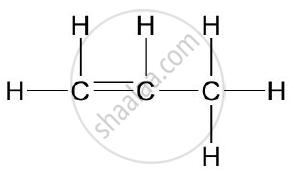Concept: Nomenclature of Organic Compounds (IUPAC)
Chapter: [0.09] Organic Chemistry

Write the IUPAC names of the following: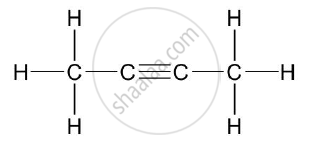Concept: Nomenclature of Organic Compounds (IUPAC)
Chapter: [0.09] Organic Chemistry

Write the IUPAC names of the following: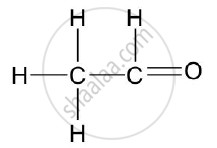Concept: Nomenclature of Organic Compounds (IUPAC)
Chapter: [0.09] Organic Chemistry

Rewrite the following sentences by using the correct symbol > (greater than) or < (less than) in the blanks given

The ionization potential of potassium is _________________ that of sodium.

Concept: Periodic Properties - Periodic Properties: Ionisation Potential (Ionisation Energy)
Chapter: [0.01] Periodic Table, Periodic Properties and Variations of Properties

Rewrite the following sentences by using the correct symbol > (greater than) or < (less than) in the blanks given

The electronegativity of iodine is ___________ that of Chlorine

Concept: Periodic Properties - Periodic Properties: Electronegativity
Chapter: [0.01] Periodic Table, Periodic Properties and Variations of Properties
 2
 2.1

Use the letters only written in the Periodic Table given below to answer the questions that Follow :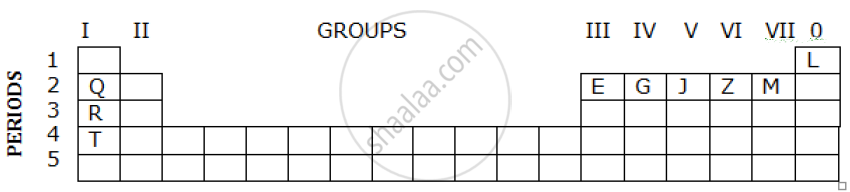1) State the number of valence electrons in atom J.

2) Which element shown forms ions with a single negative charge?

3)Which metallic element is more reactive than R?

4) Which element has its electrons arranged in four shells?

Concept: Periodic Properties
Chapter: [0.01] Periodic Table, Periodic Properties and Variations of Properties
 2.2 | Fill in the blanks by selecting the correct word from the brackets

If an element has a low ionization energy then it is likely to be ______________ (metallic / non metallic).

Concept: Types of Elements: Non-metal
Chapter: [0.07] Metallurgy

If an element has seven electrons in its outermost shell then it is likely to have the ______________ atomic size among all the elements in the same period.

Largest

Smallest

Concept: Periodic Properties - Periodic Properties: Shells (Orbits)
Chapter: [0.01] Periodic Table, Periodic Properties and Variations of Properties
 2.3

The following table shows the electronic configuration of the elements W, X, Y, Z:

 Element w x y z Electronicconfigurations 2, 8, 1 2, 8, 7 2, 5 1

Answer the following questions based on the table above:

1) What type of Bond is formed between :

a) W and X

b) Y and Z

2) What is the formula of the compound formed between :

a) X and Z

b) W and X

Concept: Periodic Properties
Chapter: [0.01] Periodic Table, Periodic Properties and Variations of Properties
 3
 3.1

Write a balanced chemical equation for Burning of ethane in the plentiful supply of air.

Concept: Ethanol
Chapter: [0.09] Organic Chemistry

Write a balanced chemical equations for Action of water on Calcium carbide

Concept: Nomenclature of Organic Compounds (IUPAC)
Chapter: [0.09] Organic Chemistry

Write a balanced chemical equations for Heating of Ethanol at 170°C in the presence of conc. Sulphuric acid

Concept: Ethanol
Chapter: [0.09] Organic Chemistry
 3.2

Give the structural formulae of 2-methylpropane.

Concept: Isomers
Chapter: [0.09] Organic Chemistry

Give the structural formulae of Ethanoic acid.

Concept: Isomers
Chapter: [0.09] Organic Chemistry

Give the structural formulae of Butan – 2 -ol

Concept: Isomers
Chapter: [0.09] Organic Chemistry
 3.3

The equation for the reaction when compound A is bubbled through bromine dissolved in carbon tetrachloride is as follows: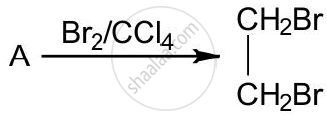1) Draw the structure of A.

2) State your observation during this reaction.

Concept: Organic Compounds
Chapter: [0.09] Organic Chemistry
 3.4

Fill in the blanks using the appropriate words given below :

(Sulphur dioxide, nitrogen dioxide, Nitric oxide, Sulphuric acid)

Cold, dilute nitric acid reacts with copper to give ___________.

Concept: Manufacture of Nitric Acid
Chapter: [0.083] Nitric Acid

Fill in the blanks using the appropriate words given below :

(Sulphur dioxide, nitrogen dioxide, Nitric oxide, Sulphuric acid)

Hot, concentrated nitric acid reacts with sulphur to form ______________.

Concept: Manufacture of Nitric Acid
Chapter: [0.083] Nitric Acid
 4
 4.1

Identify the gas evolved and give the chemical test in the following cases

Dilute hydrochloric acid reacts with sodium sulphite.

Concept: Laboratory Preparation of Hydrogen Chloride Gas
Chapter: [0.081] Hydrogen Chloride [0.1] Practical Work

Identify the gas evolved and give the chemical test in the following cases

Dilute hydrochloric acid reacts with iron (II) sulphide.

Concept: Laboratory Preparation of Hydrogen Chloride Gas
Chapter: [0.081] Hydrogen Chloride [0.1] Practical Work
 4.2

State your observations when ammonium hydroxide solution is added drop by drop and then in excess to each of the following solutions :
1) Copper sulphate solution

2) Zinc sulfate solution.

Concept: Action of Ammonium Hydroxide on Certain Salt Solutions
Chapter: [0.04] Analytical Chemistry
 4.3

Write equations for the reactions taking place at the two electrodes (mentioning clearly the name of the electrode) during the electrolysis of Acidified copper sulphate solution with copper electrodes

Concept: Electrolysis
Chapter: [0.06] Electrolysis

Write equations for the reactions taking place at the two electrodes (mentioning clearly the name of the electrode) during the electrolysis of Molten lead bromide with inert electrodes

Concept: Electrolysis
Chapter: [0.06] Electrolysis
 4.4

Name the product formed at the anode during the electrolysis of acidified water using platinum electrodes.

Concept: Electrolytes
Chapter: [0.06] Electrolysis

Name the metallic ions that should be present in the electrolyte when an article made of copper is to be electroplated with silver.

Concept: Applications of Electrolysis
Chapter: [0.06] Electrolysis
 5
 5.1

A gas cylinder contains 12 x 1024 molecules of oxygen gas.

If Avogadro’s number is 6 x 1023; Calculate:

1)The mass of oxygen present in the cylinder.

2) The volume of oxygen at S.T.P. present in the cylinder. [O = 16]

Concept: Fundamental Laws of Gases - Avogadro’s Law
Chapter: [0.05] Mole Concept and Stoichiometry
 5.2

A gaseous hydrocarbon contains 82.76% of carbon. Given that its vapor density is 29, find its molecular formula. [C = 12, H = 11]

Concept: Empirical Formula of a Compound
Chapter: [0.05] Mole Concept and Stoichiometry
 5.3

The equation 4NH3 + 5O2 → 4NO + 6 H2O, represents the catalytic oxidation of ammonia. If 100 cm3 of ammonia is used calculate the volume of oxygen required to oxidize the ammonia completely

Concept: Chemical Properties of Ammonia
Chapter: [0.08199999999999999] Ammonia
 5.4

By drawing an electron dot diagram show the formation of Ammonium ion [Atomic No.: N = 7 and H = 1]

Concept: Coordinate Bond
Chapter: [0.02] Chemical Bonding
 6
 6.1

Name the gas evolved when the following mixtures are heated:

Calcium hydroxide and Ammonium Chloride

Concept: Preparation of Aqueous Ammonia
Chapter: [0.08199999999999999] Ammonia

Name the gas evolved when the following mixtures are heated:

Sodium Nitrite and Ammonium Chloride

Concept: Laboratory Preparation of Nitric Acid
Chapter: [0.083] Nitric Acid
 6.2

Write balanced chemical equations for the following:

When the excess of ammonia is treated with chlorine.

Concept: Chemical Properties of Ammonia
Chapter: [0.08199999999999999] Ammonia

Write balanced chemical equations for the following:

An equation to illustrate the reducing nature of ammonia.

Concept: Chemical Properties of Ammonia
Chapter: [0.08199999999999999] Ammonia
 6.3

A, B, C, and D summarize the properties of sulphuric acid depending on whether it is dilute or concentrated.

A = Typical acid property

B = Non volatile acid

C = Oxidizing agent

D = Dehydrating agent

Choose the property (A, B, C or D) depending on which is relevant to each of the following

1) Preparation of Hydrogen chloride gas

2) Preparation of Copper sulphate from copper oxide.

3) An action of conc. Sulphuric acid on Sulphur.

Concept: Chemical Properties of Sulphuric Acid
Chapter: [0.084] Sulphuric Acid
 6.4

Give reasons why Sodium Chloride will conduct electricity only in the fused or aqueous solution state.

Concept: Electrolysis
Chapter: [0.06] Electrolysis

Give reasons why In the electroplating of an article with silver, the electrolyte sodium argentocyanide solution is preferred over silver nitrate solution.

Concept: Applications of Electrolysis
Chapter: [0.06] Electrolysis

Give reasons why Although copper is a good conductor of electricity, it is a non-electrolyte.

Concept: Electrolytes
Chapter: [0.06] Electrolysis
 7
 7.1

Name the solution used to react with Bauxite as a first step in obtaining pure aluminum oxide, in the Baeyer’s process

Concept: Extraction of Aluminium
Chapter: [0.07] Metallurgy

Write the equation for the reaction where the aluminum oxide for the electrolytic extraction of aluminum is obtained by heating aluminum hydroxide.

Concept: Extraction of Aluminium
Chapter: [0.07] Metallurgy

Name the compound added to pure alumina to lower the fusion temperature during the electrolytic reduction of alumina.

Concept: Reduction of Metal Oxides to Metals
Chapter: [0.07] Metallurgy

Write the equation for the reaction that occurs at the cathode during the extraction of aluminum by electrolysis.

Concept: Extraction of Aluminium
Chapter: [0.07] Metallurgy

Explain why it is preferable to use a number of graphite electrodes as the anode instead of a single electrode, during the above electrolysis.

Concept: Electrolysis
Chapter: [0.06] Electrolysis
 7.2

State what would you observe when Washing Soda Crystals are exposed to the atmosphere

Concept: Salts
Chapter: [0.03] Study of Acids, Bases and Salts

State what would you observe when The salt ferric chloride is exposed to the atmosphere

Concept: Classification of Salts
Chapter: [0.03] Study of Acids, Bases and Salts
 7.3

Identify the cations in the following case:

NaOH solution, when added to the Solution (A), gives a reddish brown precipitate

Concept: Action of Sodium Hydroxide Solution on Certain Metallic Salt Solutions
Chapter: [0.04] Analytical Chemistry

Identify the cations in the following case :

NH4OH Solution, when added to the Solution (B), gives white ppt which does not dissolve in excess.

Concept: Preparation of Aqueous Ammonia
Chapter: [0.08199999999999999] Ammonia

Identify the cations in the following case:

NaOH Solution, when added to Solution (C), gives white ppt which is insoluble in excess

Concept: Preparation of Aqueous Ammonia
Chapter: [0.08199999999999999] Ammonia

#### Request Question Paper

If you dont find a question paper, kindly write to us

View All Requests

#### Submit Question Paper

Help us maintain new question papers on Shaalaa.com, so we can continue to help students

only jpg, png and pdf files

## CISCE previous year question papers Class 10 Chemistry with solutions 2015 - 2016

CISCE Class 10 Chemistry question paper solution is key to score more marks in final exams. Students who have used our past year paper solution have significantly improved in speed and boosted their confidence to solve any question in the examination. Our CISCE Class 10 Chemistry question paper 2016 serve as a catalyst to prepare for your Chemistry board examination.
Previous year Question paper for CISCE Class 10 Chemistry-2016 is solved by experts. Solved question papers gives you the chance to check yourself after your mock test.
By referring the question paper Solutions for Chemistry, you can scale your preparation level and work on your weak areas. It will also help the candidates in developing the time-management skills. Practice makes perfect, and there is no better way to practice than to attempt previous year question paper solutions of CISCE Class 10.

How CISCE Class 10 Question Paper solutions Help Students ?
• Question paper solutions for Chemistry will helps students to prepare for exam.
• Question paper with answer will boost students confidence in exam time and also give you an idea About the important questions and topics to be prepared for the board exam.
• For finding solution of question papers no need to refer so multiple sources like textbook or guides.